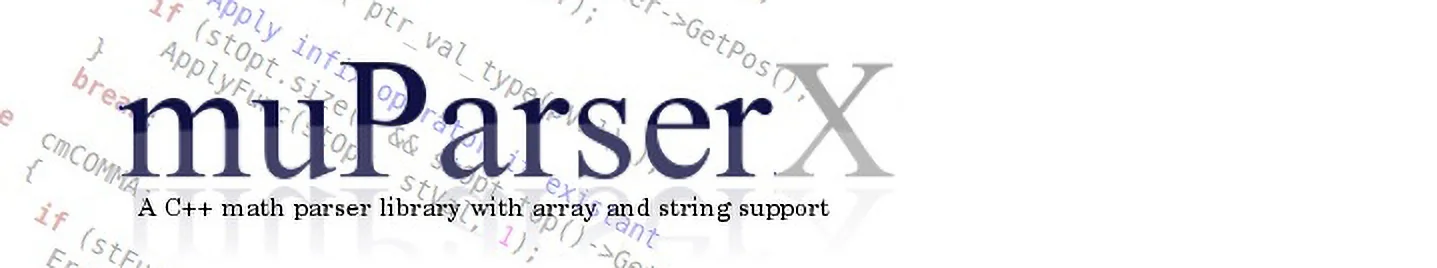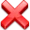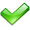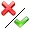# Math Parser Library

## Introduction

The evaluation of a mathematical expression is a standard task in many applications. It can be solved by using a standard math expression parser such as muparser or by embedding a scripting language such as Lua. There are however some limitations: Although muparser is pretty fast it will only work with scalar values and although Lua is very flexible it does neither support binary operators for arrays nor complex numbers. So if you need a math expression parser with support for arrays, matrices and strings muparserx may be able to help you. It was originally based on the original muparser engine but has since evolved into a standalone project with a completely new parsing engine.

Parser Data types Precision User defined operators User defined functions Localization Licence Performance (Expr. per second)
complex scalar string vector Binary Postfix Infix Strings as parameters Arbitrary number of parameters
muparser(1)doubleBSD 2-Clause ~ 10.000.000
muparserSSEfloatmax. 10BSD 2-Clause ~ 20.000.000
muparserXdoubleBSD 2-Clause ~ 1.600.000

Table 1: Feature comparison with other derivatives of muparser. (* Average performance calculated using this set of expressions; (1) muparser can define strings but only as constants.)

## Overview

• Data types: double, integer, complex, boolean, string, array
• Extensible with custom operators (binary, infix or postfix)
• Extensible with custom functions with an arbitrary number of function arguments
• Support for an unlimited number of variables and constants
• Reads binary, hexadecimal, complex, integer and string values from expressions and can be extended to read user defined values as well.
• Large variety of predefined operators, functions and constants.
• Written in standard compliant C++ code with no external dependencies.

### Predefined constants

• The eulerian number: `e = 2.718281828459045235360287`
• Pi: `pi = 3.141592653589793238462643`
• The imaginary unit with: `i = sqrt(-1)`

### Binary and ternary operators

• Standard operators: `+, -, *, /, ^`
• Assignment operators: `=, +=, -=, *=, /=`
• Logical operators: `and, or, xor, ==, !=, >, <, <=, >=`
• Bit manipulation: `&, |, <<, >>`
• String concatenation: `//`
• if then else conditionals with lazy evaluation: `?:`

### Postfix Operators

• Unit postfixes (nano, micro, milli, kilo, giga, mega): `n, mu, m, k, G, M`
• Transpose operator: `'` (for vectors and matrices)

### Infix Operators

• Sign operator and type conversions: `-, (float), (int)`

### Special Operators

• Multidimensional index operator: `a[1,2]`
• Ternary if-then-else operator: `(a<b) ? c:d`
• In-place array creation: `{1,2,3}`

### Predefined Functions

• Standard functions `abs, sin, cos, tan, sinh, cosh, tanh, ln, log, log10, exp, sqrt`
• Unlimited number of arguments: `min, max, sum`
• String functions: `str2dbl, strlen, toupper`
• Complex functions: `real, imag, conj, arg, norm`
• Array functions: `sizeof, eye, ones, zeros`

### Sample expressions

The next table shows samples of expressions that can be evaluated using muparserx: